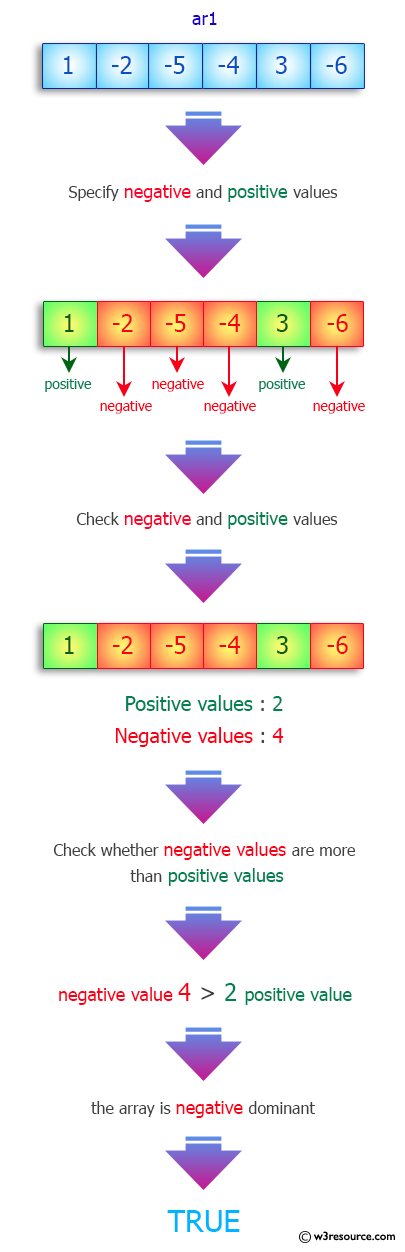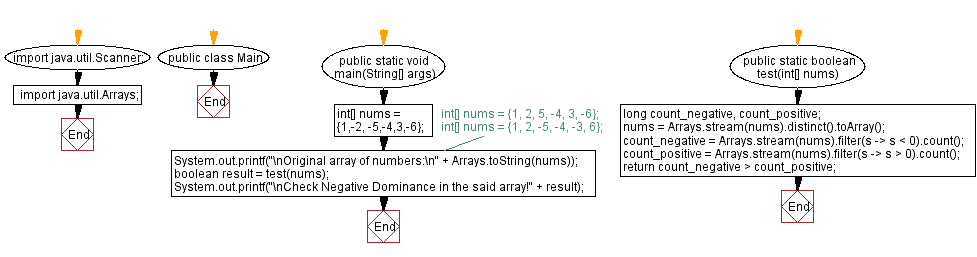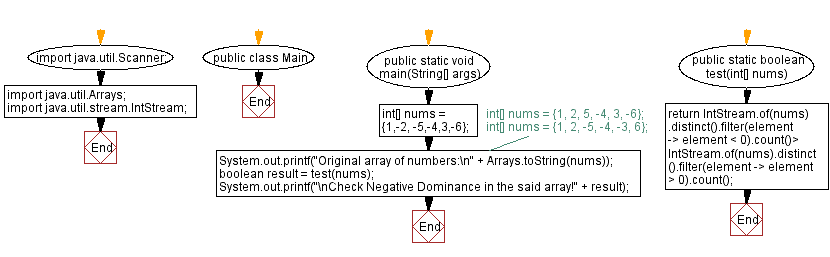﻿ Java - Negative Dominant

# Java: Negative Dominant

## Java Array: Exercise-78 with Solution

When an array has more unique negative values than unique positive values, it is negative dominant.

Write a Java program that checks whether an array is negative dominant or not. If the array is negative dominant return true otherwise false.

Sample Data:
{1,-2, -5,-4,3,-6} -> true
{1, 2, 5, -4, 3, -6} -> false
{1, 2, -5, -4, -3, 6} -> false

Pictorial Presentation:Sample Solution-1:

Java Code:

``````// Import necessary Java classes.
import java.util.Scanner;
import java.util.Arrays;

// Define the 'Main' class.
public class Main {
// Define the main method for running the program.
public static void main(String[] args) {
// Initialize an array of numbers.
int[] nums = {1, -2, -5, -4, 3, -6};
// Alternative input arrays:
// int[] nums = {1, 2, 5, -4, 3, -6};
// int[] nums = {1, 2, -5, -4, -3, 6};
System.out.printf("\nOriginal array of numbers:\n" + Arrays.toString(nums));

// Call the 'test' method to check for negative dominance in the array.
boolean result = test(nums);
System.out.printf("\nCheck Negative Dominance in the said array! " + result);
}

// Define the 'test' method to check for negative dominance in the array.
public static boolean test(int[] nums) {
long count_negative, count_positive;
// Remove duplicate values from the 'nums' array using the 'distinct' method.
nums = Arrays.stream(nums).distinct().toArray();
// Count the number of negative and positive elements in the array.
count_negative = Arrays.stream(nums).filter(s -> s < 0).count();
count_positive = Arrays.stream(nums).filter(s -> s > 0).count();
// Return 'true' if there are more negative elements, indicating negative dominance.
return count_negative > count_positive;
}
}
```
```

Sample Output:

```Original array of numbers:
[1, -2, -5, -4, 3, -6]
Check Negative Dominance in the said array!true
```

Flowchart:Sample Solution-2:

Java Code:

``````// Import necessary Java classes.
import java.util.Scanner;
import java.util.Arrays;
import java.util.stream.IntStream;

// Define the 'Main' class.
public class Main {
// Define the main method for running the program.
public static void main(String[] args) {
// Initialize an array of numbers.
int[] nums = {1, -2, -5, -4, 3, -6};
// Alternative input arrays:
// int[] nums = {1, 2, 5, -4, 3, -6};
// int[] nums = {1, 2, -5, -4, -3, 6};
System.out.printf("Original array of numbers:\n" + Arrays.toString(nums));

// Call the 'test' method to check for negative dominance in the array.
boolean result = test(nums);
System.out.printf("\nCheck Negative Dominance in the said array! " + result);
}

// Define the 'test' method to check for negative dominance in the array.
public static boolean test(int[] nums) {
// Use IntStream to process the array and check for negative dominance.
return IntStream.of(nums).distinct().filter(element -> element < 0).count() >
IntStream.of(nums).distinct().filter(element -> element > 0).count();
}
}
```
```

Sample Output:

```Original array of numbers:
[1, -2, -5, -4, 3, -6]
Check Negative Dominance in the said array!true
```

Flowchart:Java Code Editor:

Previous Java Exercise: Positive and negative values alternate in an array.
Next Java Exercise: Missing letter from an array of increasing letters.

What is the difficulty level of this exercise?

Test your Programming skills with w3resource's quiz.

﻿